PHYSICS

Mass

WAVE AND PHOTON

WA. Fundamental of Wave WB. Photon WC. Kong Vector
WE. Behaviors of Photon

WE. BEHAVIORS OF PHOTON

1. Behaviors of Photon 2. Refraction 3. Reflection 4. Diffraction
5. Interference 6. Polarity of Photon

INTRODUCTION

In this chapter, we discuss about the behavior of photon. Photon possesses the characteristic of reflection, refraction, diffraction, interference and polarity. The polarity of photon depends on the direction of magnetic and electric (M&E) components.

Here, all these characteristics are explained, described and illustrated. There is only one reason for the photon to act in such a way. It is because of the phenomenon caused by the Kong vector.

The interference fringe produced by many numbers of photons is also explained and discussed in this chapter. This solves the discrepancies on the superposition of light.

OBJECTIVES

1)      To describe and illustrate the behaviors of photon, namely refraction, reflection, diffraction, interference

and polarity.

2)      To describe the interaction between photon and mass in order to perform the behaviors.

3)      To explain the role of the Kong vector in producing all the behaviors.

4)      To explain the discrepancies on the superposition of light.

## WE.1.0        BEHAVIORS OF PHOTON

Photon possesses the characteristic or behavior of reflection, refraction, diffraction, interference and polarity. Photon is able to perform such behavior because of the structure of photon and the characteristic of photon when oscillating in different pattern of magnetic and electric (M&E) field. The Kong vector of photon is reduced when oscillating in the non-perpendicular M&E field.

In the Topic ‘Mass’, it is described that the structure of atom and mass is made up by the M&E field. An object is made up of a group of atoms. The M&E field of a mass is the superposition of all the M&E field of the atomic structure. The density of the M&E field of a mass is depending on the density of atoms. The higher the density of atoms produces the higher the density of M&E field. When the photon enters into a different pattern and density of the M&E field or the photon travels from one space to another space with different density of the M&E field, the three vectors of photon deform and react according to the surrounding. The behavior of photon is depending on the Kong vector.

The size of photon also plays important role to determine the behavior of photon. The smaller the size of photon, the higher the power of penetration of the photon.

WE.1.1        Photon Travel Medium

Photon requires a travel medium, which is the M&E field. Photon travels according to the pattern of the M&E field. To the human’s eyes and senses, a medium can be a solid object, liquid, air space or plasma. But to the photon, a medium is only the M&E field with different kinds and density of the superimposed M&E field patterns. All the objects in the world and Universe are made of the same ingredients, which is nothing but the M&E field.

WE.2.0        REFRACTION

When photon travels from one medium to another medium which have different strength and pattern of M&E field, such as a block of glass; it undergoes refraction. Glass is a transparent or porous object where the density of atom is not high. Most of the space is not filled with atom but filled with non-perpendicular M&E field. The non-perpendicular M&E field is the result of the superposition of M&E field of atoms.

In the Chapter ‘Energy of Photon’, it is described that the refraction index of medium is related to the deform angle of the M&E field of the medium. When photon enters into transparent medium, the energy of photon can be sub-divided into the kinetic and the potential energy. The M&E components of photon deform according to the M&E pattern of glass. The Kong vector of the photon changes and the traveling speed of the photon is changed too. This causes the change on the travel direction. The refraction of photon is illustrated in the figure WE.2.1.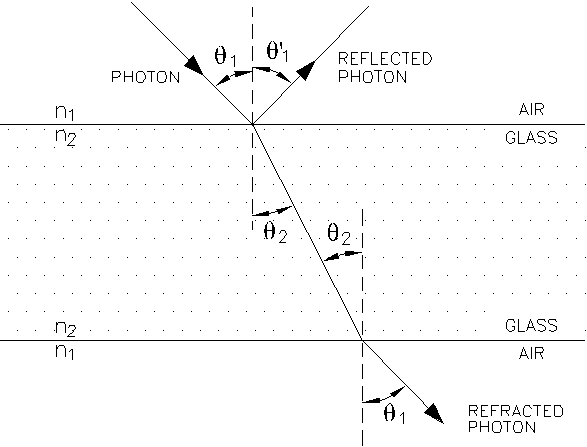Figure WE.2.1

It is first assumed that photon travels at the speed of light. Figure WE.2.1 shows that the photon passes through a block of glass. Photon is bent at the first interface due to slower traveling speed of the photon in glass medium. At the second interface, when photon leaves the block of glass, the M&E component of photon returns to the original vector; this causes the photon to change direction, back to the original direction and travel at the speed of light.

The law of refraction is derived by Huygens’ Principle on the reduced velocity of photon and wavelength. The Refraction of photon follows the law of refraction as follows,

n1 sin q1 = n2 sin q2                                                                             … eq. WE.2.1

where   n1 = c/v1 = index of refraction of medium 1

n2 = c/v2 = index of refraction of medium 2

q1 = angle of incident

q1 = angle of refraction

c = speed of photon

The refraction is due to the changes of the Kong vector and the M&E vectors of photon when entering into different pattern of the M&E field from one medium to another medium. The change on the three vectors of photon is described in figure WE.2.2.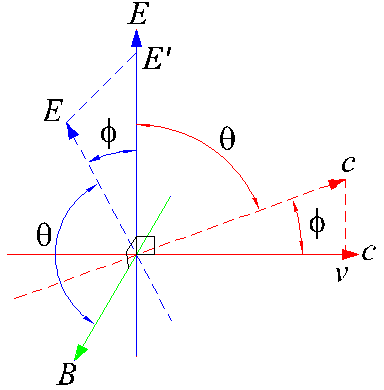Figure WE.2.2

Figure WE.2.2 shows that when the M&E component of photon is twisted, the Kong vector reduces. The reduction of the Kong vector is depending on the refraction index of the medium.

WE.3.0        REFLECTION

There are two types of reflections. The first type is due to the Kong vector. The second type is due to the collision reflection.

WE.3.1        Kong Vector Reflection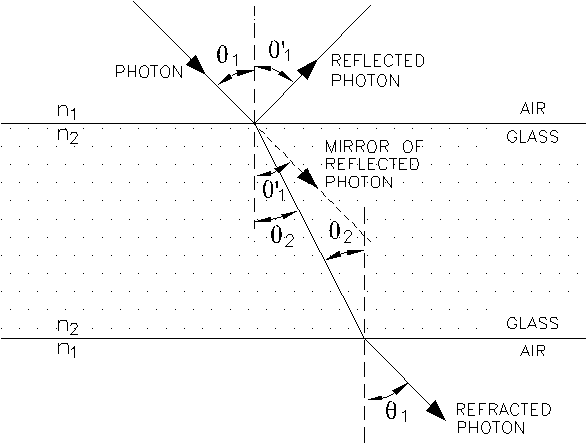Figure WE.3.1

Figure WE.3.1 shows the reflection of photon on the interface of glass. When photons hit on the first interface of glass, portion of the photons are reflected. The reflected photons made an angle to the plane of incidence, which named as angle of reflection.

The law of reflection is

q1 = q1                                                                                                … eq. WE.3.1

where   q1 = angle of incident

q1 = angle of refraction

Glass medium is a porous medium. The M&E field distribution throughout the medium is not uniform. The M&E field appears near to the atomic structure is more concentrated. From the Topic ‘Mass, we know about the structure of atom, the concentrated M&E field of atom is irregular pattern. When photons hit on the M&E field of atomic structure, the M&E vector of photons is twisted at higher angle and this causes the reverse Kong vector and the photon reflects. The photon is reflected to the same medium as the incident photon. Therefore, the velocity of photon remains.

When the photon is shot perpendicular to the glass interface, the photon is reflected at the reverse direction and normal to the glass interface. When the incident photon is not perpendicular to the glass interface, the photon is reflected to the mirror image of the incident photon.

WE.3.2        Collision Reflection

Another phenomenon that causes the reflection of photon is due to the size of photon. An object is made up of atoms. When the size of photon is bigger than the size of atom or bigger than the size of the pores between the atomic structures; where the M&E vector of photon is unable to match, squeeze and penetrate through the M&E field of the object. The collision of the photon to the medium causes the reflection. The collision may happen between the photon and the electron or the photon and the atom. In this collision reflection, the energy of photon loses to the medium.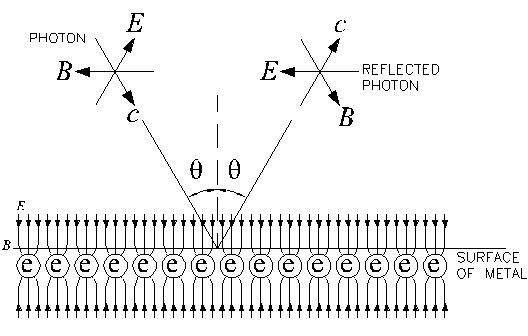Figure WE.3.2

Figure WE.3.2 shows the reflection of photons on metal surface. On the surface of certain object which has different pattern of M&E field, such as the metal surface. In the cases when the M&E component of the photon cannot match with the M&E field on the metal surface, the photon will collide on the surface and be reflected. The energy of photon may lose to the distributed electrons on the metal surface. The energy transferred heat up the metal.

WE.4.0        DIFFRACTION

WE.4.1        Diffraction Through Opening

Photon is particle-like wave-packet. The diffraction of photon is similar to the diffraction of particles. From the Chapter ‘Particles and Waves’, it is described that the diffraction of particles is caused by the quantized M&E field on the edge of the object. The similar phenomenon happens to photon. The diffraction of photon is shown in figure WE. 4.1.

Figure WE.4.1 shows the diffracted photon pattern produced when the photon passes through (A) a pin hole and (B) a slit.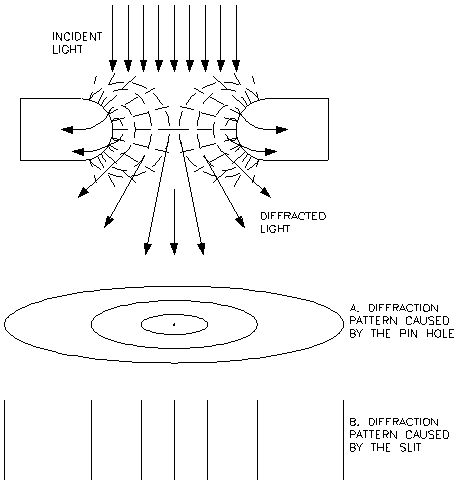Figure WE.4.1

The diffraction of photon is related to the refraction. The edge of the pin hole possesses different strength and pattern of the M&E field or the gauss line. The different strength and pattern of the M&E fields correspond to different refraction index. When the photon passes through the edge of pin hole, the photon is twisted or deformed to certain angle. The kinetic energy of photon is partially converted to the potential energy, which is also the mass property of the photon.

From the chapter “Kong Frequency and Kong Wavelength”, it is described that the diffraction of electrons and photons are related to the total energy. Both photons and electrons will be diffracted the same pattern when they possess the same energy level. The diffraction of electron incident beam is due to the reaction of electrons to the strong M&E fields near the edge of the pin hole. This diffraction phenomenon is named the Kong Effect. The diffraction of electrons incident beam is described in the chapter "Particles and Waves".

From chapter “energy of photon”, the potential energy or the mass property of the photon is given as,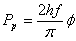… eq. WD.4.1

The diffraction pattern is depending on the Mass Effect. The mass property is depending on the frequency of the photon. For the same size of pin hole with the same refraction index, the deform angle is the same. The diffraction pattern is only depending on the frequency of the incident photon.

The outer edge of the pin hole possesses weaker M&E field, the deform angle is minimal, the mass property of photon is minimal and the diffracted angle is also minimal. It produces the first maximal of the diffraction pattern.

The inner edge of the pin hole possesses stronger M&E field, the deform angle is maximal, the mass property of photon is maximal and the diffracted angle is also maximal. It produces the second and higher maximal of the diffraction pattern.

The same principle applies to the diffraction through the slit. The outer edge of slit possesses weaker M&E field and stronger at the inner edge. The diffraction of photon through the slits is also shown in figure WE.4.1.

The diffraction pattern is also depending on the size of photon. If the size of the hole is smaller than the size of photon, the photon cannot penetrate through the hole. If the size of the photon is smaller than the pin hole, the photon can penetrate through the hole and be diffracted.

Different frequency of photon has different size and different energy level. Higher frequency photon possesses smaller size. Smaller size of photon deforms to smaller size of mass. Smaller size of photon can fit and distinguish better on the discrete magnetic gauss line and produce sharper pattern. Higher energy level of photon is diffracted at smaller tangential angle and produces smaller ring.

The diffraction of photon adheres to the law of diffraction. For the same size of opening, the smaller size of photon has shorter wavelength and higher frequency; the distance between each maximal of diffraction pattern is shorter. It is vice versa for bigger size of photon.

Electron incident beam produces the diffraction pattern bases on the same principle. This is explained in the Chapter ‘Particles and Waves’.

WE.4.2        Diffraction on Crystal

The principle about the diffraction of photon on crystal structure is similar to the refraction of photon through glass block. Glass is a porous structure with random atomic arrangement, while crystal possesses certain pattern of well arranged crystal structure.

X-ray possesses the smaller size of photon compare to visible light. X-ray has the higher power in distinguishing the M&E field. X-ray is able to penetrate through the crystal structure which the distance of each atom is about the x-ray wavelength. When x-ray penetrates the empty space between atoms, it is refracted. When x-ray hits on the concentrated M&E field of atoms at deeper layers, x-ray is reflected. The reflected x-ray undergoes refraction when passes through the crystal medium and then leaves the crystal structure. The refracted and reflected x-ray produces the diffraction pattern on the collecting target.

WE.4.3        Interference Fringe

When photon passes through a slit, diffraction happened. When the same source of photons passes through two or more identical slits, diffraction happened on every slit. When the slits are adjusted so that each diffracted photons from each slit falls on the same position of target, the superimposed diffraction pattern is produced. This pattern is called interference fringe. Figure WE.4.2 shows the interference fringe produced by two identical slits.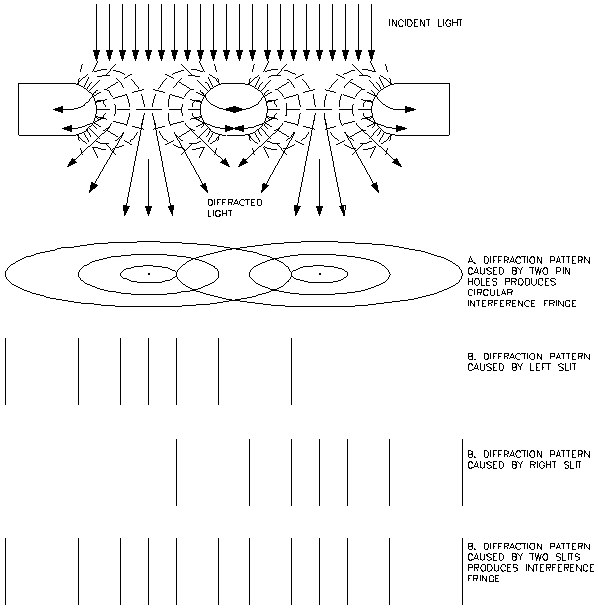Figure WE.4.2

The intensity distribution of the interference fringe depends on the intensity of the incident photon. In cases when the incident photon has the uniform intensity, the interference fringe produced follows the same intensity profile of the incident photon. In cases when the incident photon has the bell shape, the interference fringe produced a general bell shape.

WE.4.4        Discrepancies on Interference Fringe

Interference of light was previously understood that it is the phenomenon of two sources of coherent light undergoes constructive and destructive superposition and produces interference fringes on the collecting target. This is always referring to the similar interference produced by water wave.

However, recently, many experiments found that when photon is shot one photon by one to a twin slit, the collected profile after number of photons shows interference fringe too. In this case, constructive and destructive superposition could not be happened.

The same principle applies to the electron incident beam. When electron is shot one by one from the same source, passes through the slits and collected on the target, the collected electrons profile also produces interference fringe. But, the reason for the possibility of electrons to produce constructive and destructive superposition is still unresolved.

In these two cases, the only reason is the photons and electrons do not undergo constructive or destructive superposition which was adopted previously. The interference fringe produced by photons and electrons is caused by the multiple diffractions.

WE.4.5        Conservation of Momentum for Interference Fringe

Interference fringe is the phenomenon of the few diffraction patterns produced on the target when the photons pass through two or more slits. Figure WE.4.3 below shows the phenomenon of producing the interference fringe.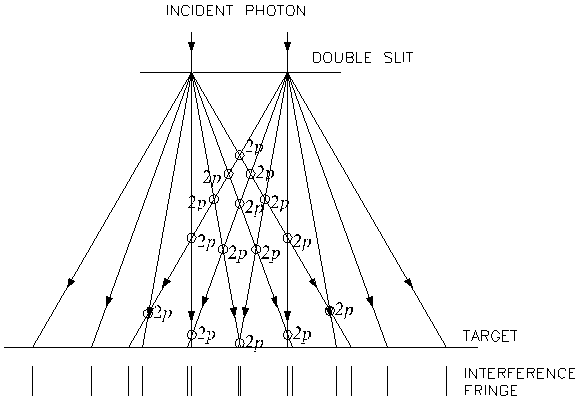Figure WE.4.3

Each diffracted beam possesses the same momentum. When the two or more diffracted beams meet, the superimposed momentum is the total summation of the momentum of each beam. The principle of momentum conservation is adhered.

In the case where the minimal interference fringe produced, the summation of momentum shall never be zero. Once again, the principle on the construction and destructive superposition of photon is deceived.

WE.5.0        INTERFERENCE

The interference discussed here is quite similar to the interference happen to the electronic equipment, where the signal received by electronic equipment is disturbed by other EM wave sources.

The interference of photon happens when two photons with difference frequencies travel together. Photons at different frequency possess different sizes. Figure WE.5.1 (A) shows the interference of two photons of difference frequencies. Two photons are traveling at the same velocity, but oscillating at difference frequencies. The M&E components of two photons will interfere or ‘collide’ with each other. This causes them to diffract away from each other and therefore cannot be focused.

Figure WE.5.1 (B) shows two monochromatic photons travel together. When photons at the same frequency travel together, they can be highly focused. It is because the M&E components of the two photons oscillate harmonically without interfering each other. Laser is a monochromatic photon and therefore can be highly focused.

The interference of photons causes the intra-diffraction between photons. When photons propagate from a point source, the photons interfere, collide and spread to a bigger surface area.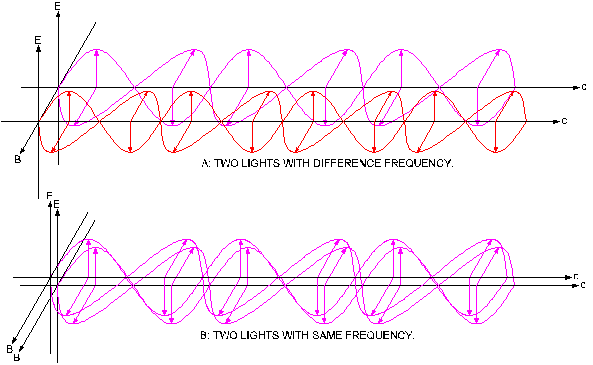Figure WE.5.1

WE.6.0        POLARITY OF PHOTON

Photon possesses M&E components. The M&E components are perpendicular to each other when travels at the speed of light. At certain frequency of photon, the M&E components are fixed. It is because each component creates each and maintains the existence of each component.

When photon passes through the external M&E fields, the M&E components of photon will be rotated to match with the external M&E fields. When photon passes through a slit or longitudinal opening, the M&E components of photon will be induced and arranged according to the M&E field of the surrounding. This phenomenon is called polarization.

Figure WE.6.1 shows the polarization of photons when passes through a slit. Before entering into the slit, the direction of the M&E components of photon can be at any angles. When the photon passes through the slit, the magnetic component of the photon is arranged into or outward on the plane while the electrical component is pointing right or left on the plane. The photons are then traveling at one pattern and direction after passes through the slit.

The same principle applied to the polarizing sheet. When photon passes through the polarizing sheet, the M&E component of photon is arranged and produces the polarizing effect.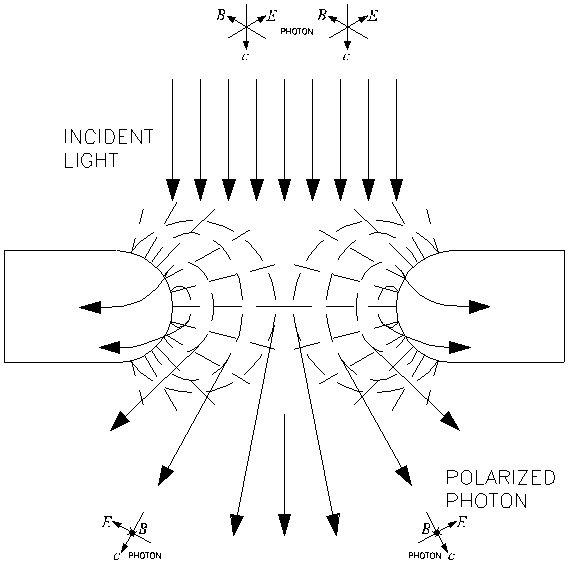Figure WE.6.1

CONCLUSIONS

Photon possesses the characteristic of reflection, refraction, diffraction, interference and polarity. These behaviors are caused by the reaction of the M&E and the Kong vector.

When photon enters into a medium with different pattern of M&E field, the photon is refracted. When photon is unable to penetrate the medium, it will be reflected.

The diffraction of photons is because of the appearance of the quantized M&E field near the edge of the openings.

Interference fringe is the effect of the multiple diffractions.

Interference of photons causes the photons at different frequencies unable to focus and to spread to a bigger surface area.

This website is originated on 15-Mar-2007,

updated on 4-Jan-2009.